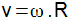Mechanics

# Main equations of kinematics

Kinematics is part of the studies of Mechanics and is the branch of Physics that studies the movement itself, without paying attention to its causes. We have gathered for you the main equations seen in this branch!

Mechanics is the branch of physics that studies motion. Within Mechanics, there is the mathematical study of movements, which is called Kinematics , in which the movement itself is the object of study, and not its causes. The causes of movement are studied by Dynamics.

Here are the main equations of kinematics:

1) Average speed: it is the result of the ratio between the space traveled by a piece of furniture and the time spent.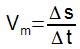2) For uniform rectilinear motion (MRU), in which the velocity of the mobile is constant, we have:

→ Speed ​​time function: indicates the position of the furniture over time.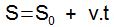For this function, we can write:

S = Final position of the furniture;
0 = Initial position of the furniture;
v = Speed;
t = Time.

3) Acceleration : vector quantity that determines the rate of change in velocity. It is given by the ratio of the change in velocity to time.4) For uniformly varied motion (MRUV), we have:

→ Speed ​​time function : provides the speed value at a given time.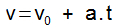For this function, we can write:

v = Final speed of the mobile;
0 = Initial speed of the mobile;
a = Acceleration;
t = Time.

→ Position time function for the MRUV: determines the position of the furniture that performs uniformly varied rectilinear movement.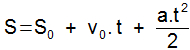For this function, we can write:

S = Final position of the furniture;
0 = Initial position of the furniture;
0 = Initial speed of the mobile;
a = Acceleration;
t = Time.

→ Torricelli’s Equation : Determines the final velocity of a mobile without the need for time.For this equation, we can write:

v = Final speed of the mobile;
0 = Initial speed of the mobile;
a = Acceleration;
Δs = Position variation.

5) For uniform circular motion (MCU), we have:

→ Angular velocity (ω ) : Result of the ratio between the angular displacement of an object and time.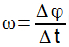→ Relationship between scalar speed (ω) and linear speed (v):